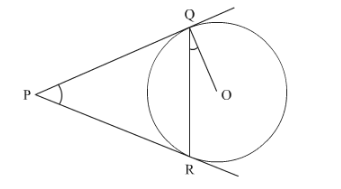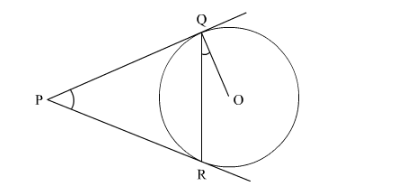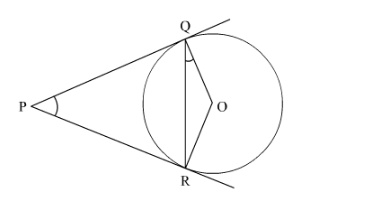# In Fig. 3, two tangents PQ are PR are drawn to a`
Question:

In Fig. 3, two tangents PQ are PR are drawn to a circle with centre O from an external point P. Prove that ∠QPR = 2 ∠OQR.Solution:Given a figure as shown. We have to prove thatJoin ORWe know that sum of opposite angles of a cyclic quadrilateral$\angle Q O R=180^{\circ}-\angle Q P R$

In $\triangle O Q R$

$O Q=O R$ [Since $O Q, O R$ are radii of the circle]

Therefore $\triangle O Q R$ is an isosceles triangle.

Hence[Angles opposite to equal side of isosceles triangle]

Now, $\angle O Q R=\frac{1}{2}\left(180^{\circ}-\angle Q O R\right)$ [since, $\angle O Q R=\angle O R Q$ ]

$\angle O Q R=\frac{1}{2}\left(180^{\circ}-\left(180^{\circ}-\angle Q P R\right)\right)$ [From (1)]

$\angle O Q R=\frac{1}{2}(\angle Q P R)$

$\angle Q P R=2 \angle O Q R$

Hence proved.